﻿ Principle of Waveplate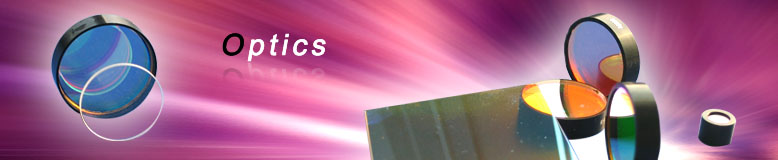OpticsWaveplatePrinciple of Waveplate
 Waveplates (retardation plates or phase shifters) are made from materials which exhibit birefringence. The velocities of the extraordinary and ordinary rays through the birefringent materials vary inversely with their refractive indices. The difference in velocities gives rise to a phase difference when the two beams recombine. In the case of an incident linearly polarized beam this is given by a=2pi*d(ne-no)/l (a-phase difference; d-thickness of waveplate; ne,no-refractive indices of extraordinary and ordinary rays respectively; l-wavelength). At any specific wavelength the phase difference is governed by the thickness of the retarder.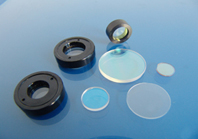Transmission range:330nm-2100nm Thermal Expansion Coefficient:7.5x10-6/K .Density:2.51g/cm3 Half Waveplate The thickness of a half waveplate is such that the phase difference is l/2-wavelength (true-zero order) or some multiple of l/2-wavelength (multiple order). A linearly polarized beam incident on a half waveplate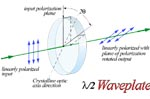emerges as a linearly polarized beam but rotates such that its angle to the optical axis is twice that of the incident beam. Therefore, half waveplates can be used as continuously adjustable polarization rotators. Half waveplates are used in rotating the plane of polarization, electro-optic modulation and as a variable ratio beamsplitter when used in conjunction with a polarization cube. Quarter Waveplate The thickness of the quarter waveplate is such that the phase difference is l/4 wavelength (true-zero order) or some multiple of l/4 wavelength (multiple order). If the angle q (between the electric field vector of the incident linearly polarized beam and the retarder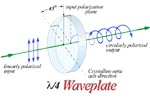principal plane) of the quarter waveplate is 45, the emergent beam is circularly polarized. When a quarter waveplate is double passed, i.e. by mirror reflection, it acts as a half waveplate and rotates the plane of polarization to a certain angle. Quarter waveplates are used in creating circular polarization from linear or linear polarization from circular, ellipsometry, optical pumping, suppressing unwanted reflection and optical isolation. Order Information: Part No.+Retardance+Wavlength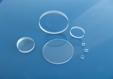Sample: WPL1101+ l /2+1064nm